# atmosphere

## Syntax

atmos = atmosphere(scenario,model)
atmos = atmosphere(___,Name,Value)

## Description

example

atmos = atmosphere(scenario,model) creates an atmosphere object atmos that belongs to a radar scenario object. The atmosphere is defined by the atmospheric refraction model. To enable this object method, set the IsEarthCentered property of the radarScenario object scenario to true. Use this object only in a scenario that uses a radarTransceiver object.After creating the atmosphere, you can use the effearthradius object function to compute the effective earth radius and the effective earth radius factor.

example

atmos = atmosphere(___,Name,Value) sets the atmosphere object with additional properties specified by one or more name-value arguments.

## Examples

collapse all

Create an atmosphere using the effective earth radius model. Assume the effective earth radius is 4/3 of the actual earth radius. Using the model requires that the IsEarthCentered property of radarScenario be true.

scenario = radarScenario('IsEarthCentered',true); atmos = atmosphere(scenario,'EffectiveEarth')
atmos = AtmosphereEffectiveEarth with properties: InputFormat: 'Radius' EffectiveEarthRadius: 8.4774e+06 

Compare the effective Earth factors calculated from the CRPL and 4/3 Earth models. Assume the slant range is 100 km, the antenna heights range from 1 to 10 km, and the target altitude is at the surface.

 SR = 100e3; ha = linspace(1,10,50).*1e3; ht = 0;

Create a radar scenario and a CRPL atmosphere.

scenario = radarScenario('IsEarthCentered',true); atmos = atmosphere(scenario,'CRPL'); [~,kCRPL] = effearthradius(atmos,SR,ha,ht);

Plot the computed k-factor and a vertical 4/3 line.

semilogy(kCRPL,ha*1e-3) hold on xline(4/3,'-.r') xline(1,'--k') xlim([0.99 1.37]) grid on legend('CRPL','4/3 Earth','True Earth') xlabel('Effective Earth Radius Factor k') ylabel('Altitude (km)') hold off## Input Arguments

collapse all

Radar scenario, specified as a radarScenario object.

Atmospheric model, specified as 'FreeSpace', 'EffectiveEarth', 'RefractivityGradient', or 'CRPL'.

Data Types: char | string

### Name-Value Arguments

Specify optional pairs of arguments as Name1=Value1,...,NameN=ValueN, where Name is the argument name and Value is the corresponding value. Name-value arguments must appear after other arguments, but the order of the pairs does not matter.

Example: InputFormat='k Factor'

No additional name-value pairs are required when the model argument is set to FreeSpace.

EffectiveEarth model

collapse all

Input format, specified as 'Radius' or 'k Factor'. Setting the InputFormat property to 'Radius' enables the EffectiveEarthRadius property. Setting the InputFormat property to 'k Factor' enables the EffectiveEarthK property.

#### Dependencies

Data Types: char | string

Effective earth radius, specified as a positive scalar. The default earth radius of 4/3 Re is equivalent to a refractivity gradient of -39e-9. Re is the radius of the earth and is obtained from physconst('EarthRadius'). Units are in meters.

.

Example: 11/7 Re

#### Dependencies

To enable this property, set the model argument to 'EffectiveEarth' and set the InputFormat property to 'Radius'.

Data Types: double

Effective earth k-factor, specified as a nonnegative scalar. The effective Earth radius Re in this case is calculated as: Re = k*Rearth, where Rearth is the Earth radius as output by physconst('EarthRadius').

Example: 11/7

#### Dependencies

To enable this property, set the model argument to 'EffectiveEarth' and set the InputFormat property to 'k Factor'.

Data Types: double

collapse all

Refractivity gradient, specified as a scalar. The refractivity gradient is used to calculate the effective Earth radius.

#### Dependencies

To enable this property, set the model argument to 'RefractivityGradient'.

Data Types: double

CRPL

collapse all

Surface refractivity, specified as a non-negative scalar. Units are N-units.

#### Dependencies

To enable this property, set the model argument to 'CRPL'.

Data Types: double

Refraction exponent factor for the CRPL exponential reference atmosphere model, specified as a non-negative scalar. Units are 1/km.

#### Dependencies

To enable this property, set the model argument to 'CRPL'.

Data Types: double

Maximum number of iterations for the CRPL method, specified as a non-negative, scalar integer. This input acts as a safeguard to prevent endless iterative calculations.

Example: 20

#### Dependencies

To enable this property, set the model argument to 'CRPL'.

Data Types: double

Specifies the absolute tolerance for the CRPL method, specified as a positive scalar. This is the tolerance which the iterative process is terminated.

Example: 0.1

#### Dependencies

To enable this property, set the model argument to 'CRPL'.

Data Types: double

## Output Arguments

collapse all

Atmosphere, returned as an Atmosphere object.

collapse all

### Curved Earth Model

The fact that the index of refraction of air depends on height can be treated approximately by using an effective Earth's radius larger than the actual value.

Given the effective Earth's radius R0, the antenna height ha, and the initial elevation angle θ0, the model relates the target height hT and the slant range RT by

${\left({R}_{0}+{h}_{T}\right)}^{2}={\left({R}_{0}+{h}_{a}\right)}^{2}+{R}_{T}^{2}+2{R}_{T}\left({R}_{0}+{h}_{a}\right)\mathrm{sin}{\theta }_{0},$

so knowing one of those magnitudes enables you to compute the other. In particular,

${h}_{T}=\sqrt{{\left({R}_{0}+{h}_{a}\right)}^{2}+{R}_{T}^{2}+2{R}_{T}\left({R}_{0}+{h}_{a}\right)\mathrm{sin}{\theta }_{0}}-{R}_{0}.$

The actual range R is equal to the slant range. The true elevation angle θT is equal to the initial elevation angle.

To compute the ground range G, use

$G={R}_{0}\varphi ={R}_{0}\mathrm{arcsin}\frac{{R}_{T}\mathrm{cos}{\theta }_{0}}{{R}_{0}+{h}_{T}}.$A standard propagation model uses an effective Earth's radius that is 4/3 times the actual value. This model has two major limitations:

1. The model implies a value for the index of refraction near the Earth's surface that is valid only for certain areas and at certain times of the year. To mitigate this limitation, use an effective Earth's radius based on the near-surface refractivity value.

2. The model implies a value for the gradient of the index of refraction that is unrealistically low at heights of around 8 km. To partially mitigate this limitation, use an effective Earth's radius based on the platform altitudes.

For more information, see effearthradius.

### CRPL Exponential Reference Atmosphere Model

Atmospheric refraction evidences itself as a deviation in an electromagnetic ray from a straight line due to variation in air density as a function of height. The Central Radio Propagation Laboratory (CRPL) exponential reference atmosphere model treats refraction effects by assuming that the index of refraction n(h) and the refractivity N decay exponentially with height. The model defines

$N=\left(n\left(h\right)-1\right)×{10}^{6}={N}_{\text{s}}{e}^{-{R}_{\text{exp}}h},$

where Ns is the atmospheric refractivity value (in units of 10–6) at the surface of the earth, Rexp is the decay constant, and h is the height above the surface in kilometers. Thus

$n\left(h\right)=1+\left({N}_{\text{s}}\text{\hspace{0.17em}}×{10}^{-6}\right){e}^{-{R}_{\text{exp}}h}.$

The default value of Ns is 313 N-units and can be modified using the SurfaceRefractivity name-value argument in functions that accept it. The default value of Rexp is 0.143859 km–1 and can be modified using the RefractionExponent name-value argument in functions that accept it.

### CRPL Model Geometry

When the refractivity of air is incorporated into the curved Earth model, the ray paths do not follow a straight line but curve downward. (This statement assumes standard atmospheric propagation and nonnegative elevation angles.) The true elevation angle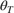is different from the initial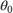. The actual range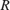, which is the distance along the curved path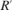, is different from the slant range.

Given the Earth's radius, the antenna height, the initial elevation angle, and the height-dependent index of refractionwith value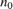at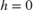, the modified model relates the target heightand the actual rangebyWhen Method is specified as "CRPL", the integral is solved usingfrom CRPL Exponential Reference Atmosphere Model.

To compute the ground range, use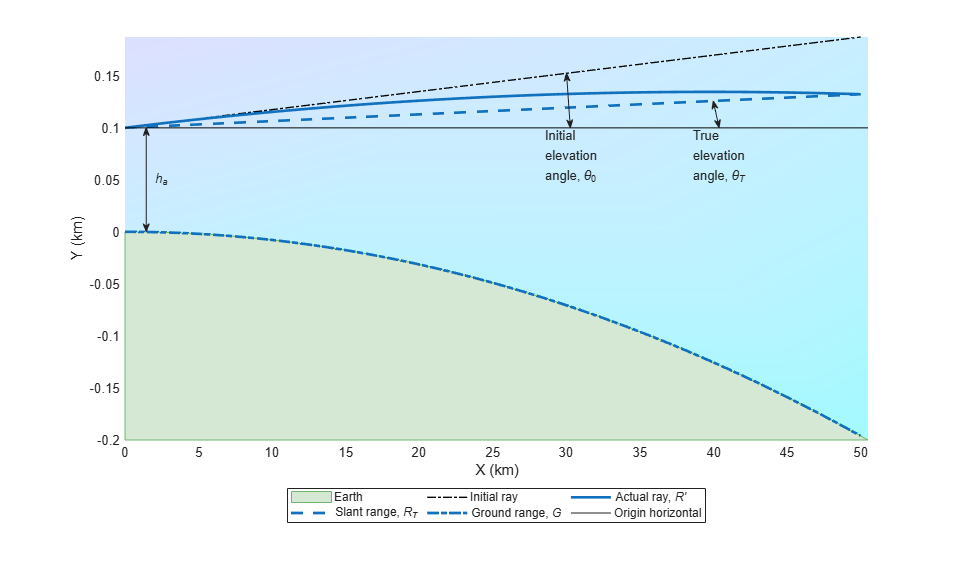### N-units

N-units are a convenient way to express the index of refraction. Because the index of refraction is very close to unity, N-units express just the deviation from unity. The refractivity N in N-units is related to the index of refraction n by

$N=\left(n-1\right)×{10}^{6}\text{.}$

For example, an index of refraction of 1.000313 becomes 313 in N-units. N-units are dimensionless.

 Bradford R. Bean, G. D. Thayer. CRPL Exponential Reference Atmosphere, U.S. Department of Commerce, National Bureau of Standards, 1959.

 Blake, L. V. "A Note on Selection of an Atmospheric Refractivity Model for Radar Range-Height-Angle Charts." NRL Report 5626, Apr. 24, 1961

 Blake, L.V. "Ray Height Computation for a Continuous Nonlinear Atmospheric Refractive-Index Profile." RADIO SCIENCE, Vol. 3 (New Series), No. 1, Jan. 1968, pp. 85-92.

 Doerry, A. W. "Correcting Radar Range Measurements for Atmospheric Propagation Effects." edited by Kenneth I. Ranney and Armin Doerry, 90771K. Baltimore, Maryland, USA, 2014. https://doi.org/10.1117/12.2048977.

 Doerry, A. W. "Earth Curvature and Atmospheric Refraction Effects on Radar Signal Propagation." Sandia National Laboratories, SAND2012-10690, Jan. 2013.

 Robertshaw, G. "Effective Earth Radius for Refraction of Radio Waves at Altitudes above 1 Km." IEEE Transactions on Antennas and Propagation 34, no. 9 (September 1986): 1099-1105. https://doi.org/10.1109/TAP.1986.1143948.

 Sweezy, W. B. , and B. R. Bean. "Correction of Atmospheric Refraction Errors In Radio Height Finding." Journal of Research of the National Bureau of Standards, D. Radio Propagation, 67D, no. 2 (March - April 1963).